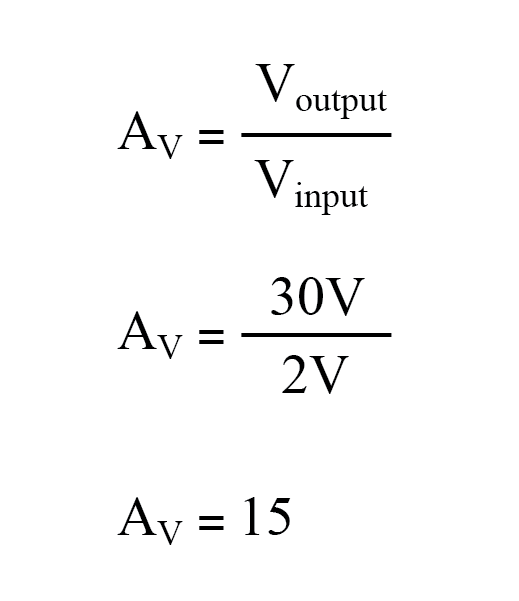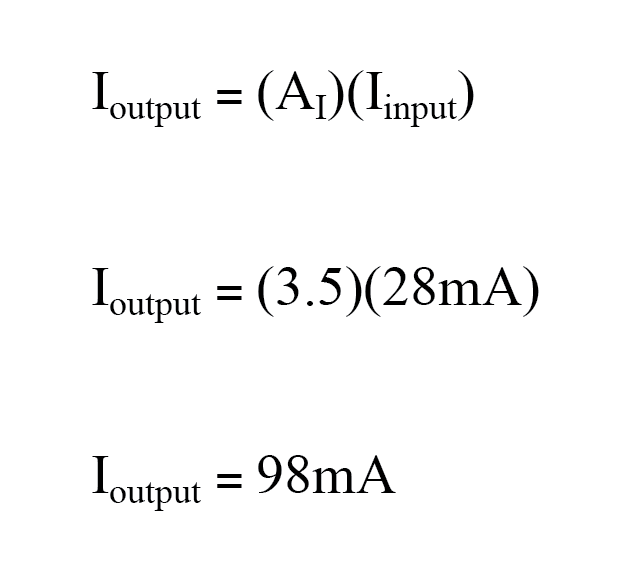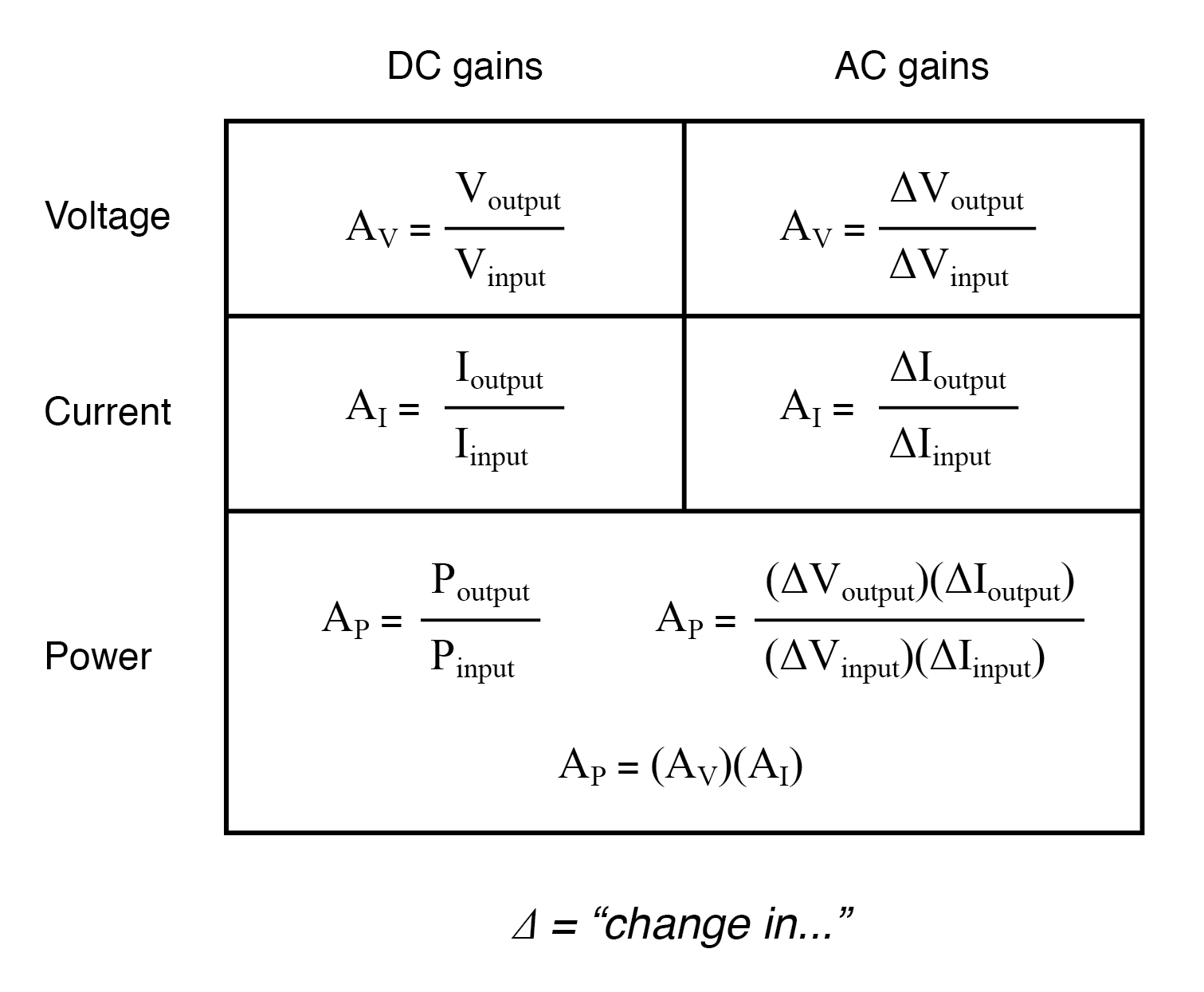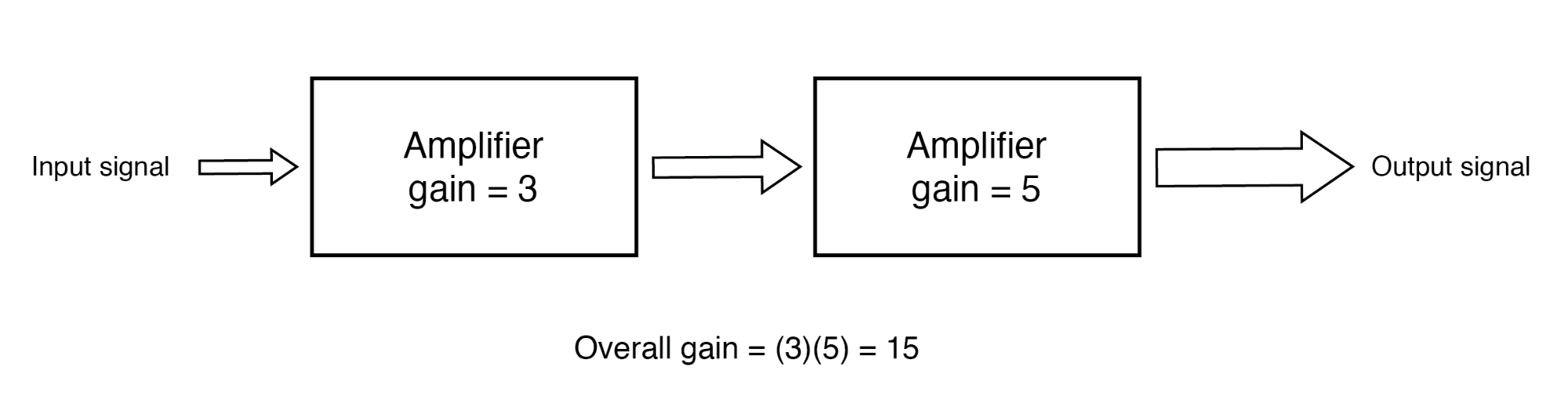# Amplifier Gain

## Chapter 1 - Amplifiers and Active Devices

### The Voltage Gain

Because amplifiers have the ability to increase the magnitude of an input signal, it is useful to be able to rate an amplifier’s amplifying ability in terms of an output/input ratio. The technical term for an amplifier’s output/input magnitude ratio is gain. As a ratio of equal units (power out / power in, voltage out / voltage in, or current out / current in), gain is naturally a unitless measurement.

Mathematically, gain is symbolized by the capital letter “A”.

### How to Calculate Voltage Gain

For example, if an amplifier takes in an AC voltage signal measuring 2 volts RMS and outputs an AC voltage of 30 volts RMS, it has an AC voltage gain of 30 divided by 2, or 15:Correspondingly, if we know the gain of an amplifier and the magnitude of the input signal, we can calculate the magnitude of the output. For example, if an amplifier with an AC current gain of 3.5 is given an AC input signal of 28 mA RMS, the output will be 3.5 times 28 mA or 98 mA:In the last two examples, I specifically identified the gains and signal magnitudes in terms of “AC”. This was intentional, and illustrates an important concept: electronic amplifiers often respond differently to AC and DC input signals and may amplify them to different extents.

Another way of saying this is that amplifiers often amplify changes or variations in input signal magnitude (AC) at a different ratio than steady input signal magnitudes (DC). The specific reasons for this are too complex to explain at this time, but the fact of the matter is worth mentioning.

If gain calculations are to be carried out, it must first be understood what type of signals and gains are being dealt with, AC or DC.

### Electrical Amplifier Gains: Voltage, Current, and/or Power

Electrical amplifier gains may be expressed in terms of voltage, current, and/or power in both AC and DC.

A summary of gain definitions is as follows:

The triangle-shaped “delta” symbol (Δ) represents change in mathematics, so “ΔVoutput / ΔVinput” means “change in output voltage divided by change in input voltage,” or more simply, “AC output voltage divided by AC input voltage”:If multiple amplifiers are staged, their respective gains form an overall gain equal to the product (multiplication) of the individual gains. In the figure below, if a 1 V signal were applied to the input of the gain of 3 amplifier, a 3 V signal out of the first amplifier would be further amplified by a gain of 5 at the second stage yielding 15 V at the final output.The gain of a chain of cascaded amplifiers is the product of the individual gains.

RELATED WORKSHEETS: